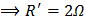# Two cells having emf 4V, 2V and internal resistances 11are connected as shown in figure below. Current through 6resistance isa)b)c) 1A d)## Question ID - 150219 :- Two cells having emf 4V, 2V and internal resistances 11are connected as shown in figure below. Current through 6resistance isa)b)c) 1A d)3537

 (a) The emf of the circuit isIn the given circuit, 3and 6are connected in parallel, hence equivalent resistance isTotal resistance of circuit isFrom Ohm’s lawThe 3resistors are in parallel, henceKirchhoff’s first lawat a junction is based on the law of conservation of a) Charge b) Energy c) Momentum d) Angular momentum